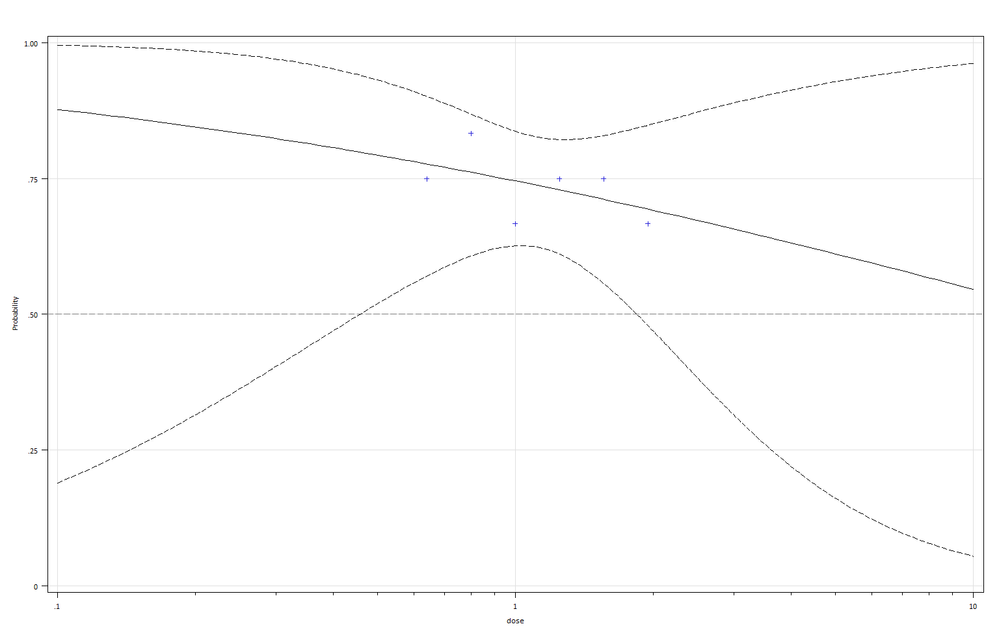## Probit analysis for LD50 calculation

I am trying to do probit analysis for 95% confidence Limit and it is my first time to use this procedure.

I've got an example in .xls file which is downloaded from https://probitanalysis.wordpress.com/2016/07/07/first-blog-post/.

I used codes as following (reference to LD50 calculation for mixture of dose concentrations in community😞

`data A;input dose total dead;cards;0.03 10 00.10 10 10.30 10 53.00 10 8;proc probit data=A log10 plot=predpplot;model Dead / Total = dose / d=logistic InverseCL(prob=0.1 to 0.9 by 0.1);run;`

However, the results of 95% Fiducial Limits are different from example and proc probit dose not provide information for curve fitting ( I need to use proc reg?). How can I reproduce the result from the example file?

Furthermore, I used the same code for my data;

``````data B;
cards;
0.64 12 9
0.8 12 10
1 12 8
1.25 12 9
1.56 12 9
1.95 12 8
;

proc probit data=B log10 plot=predpplot;
model dead / total = dose / d=logistic  InverseCL(prob=0.1 to 0.9 by 0.1);
run;``````

The 95% Fiducial Limits didnot estimate in the result and there was no warring from SAS. I am wondering if I could find any suggestion or solution for my data.

1 ACCEPTED SOLUTION

Accepted SolutionsPGStats
Opal | Level 21

## Re: Probit analysis for LD50 calculation

Look at the (traditional graphics) graph produced by :

``````data B;
cards;
0.64 12 9
0.8 12 10
1 12 8
1.25 12 9
1.56 12 9
1.95 12 8
;

proc probit data=B log10 plot=none;
model dead / total = dose / d=logistic  InverseCL(prob=0.1 to 0.9 by 0.1);
predpplot vref=0.5;
run;``````LD50 is so far from the data range and the confidence interval so large that they would be meaningless.

PG
2 REPLIES 2PGStats
Opal | Level 21

## Re: Probit analysis for LD50 calculation

Look at the (traditional graphics) graph produced by :

``````data B;
cards;
0.64 12 9
0.8 12 10
1 12 8
1.25 12 9
1.56 12 9
1.95 12 8
;

proc probit data=B log10 plot=none;
model dead / total = dose / d=logistic  InverseCL(prob=0.1 to 0.9 by 0.1);
predpplot vref=0.5;
run;``````LD50 is so far from the data range and the confidence interval so large that they would be meaningless.

PG

Hi, PG